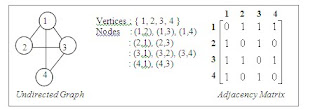/* related posts with thumb nails */

###Representation of a Graph:

Graphs can be represented using matrices. Adjacency matrix is n x n matrix A. Each element of ‘A’ is either zero or one.

Assumptions:

 G Graph V Set of Vertices (i, j) Edge connecting Vi and Vj A[i][j] Adjacency matrix element i,j VerticesExample:

Consider the following graph.

The graph has 4 vertices and it is undirected graph. Write 1 to Number of vertices i.e. 1 to 4 as row and column headers to represent it in adjacency matrix.

If edge exists between any two nodes (row, column headers indicate nodes) write it as 1 otherwise 0 in the matrix.Related Topics: